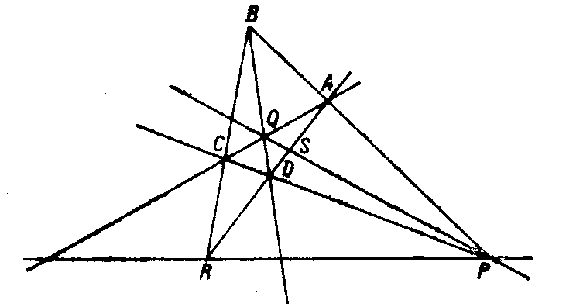##### Actions

A collection of four points $A,B,C,D$ (lying in a plane), no three of which lie on the same line, and the six lines connecting these points (cf. Fig.).The points $A,B,C,D$ are called the vertices, and the lines $AB,CD,AC,BD,BC,AD$ are called the edges of the complete quadrangle. Edges that have no common vertex are called opposite; the points $P,Q,R$ of intersection of the opposite edges are called diagonal points.
If $S$ and $T$ are the points of intersection of the line $PQ$ with the lines $AD$ and $BC$, then the four points $P,Q,S,T$ form a harmonic quadruple of points. The dual figure to a quadrangle is called a quadrilateral — a collection of four lines (in a plane), no three of which contain a common point.